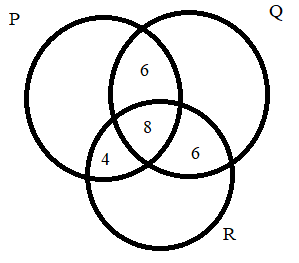Home/Class 11/Maths/

In a survey it was found that $$21$$ people liked product $$A, 26$$ liked product $$B$$ and $$29$$ liked product $$C$$. If $$14$$ people liked products $$A$$ and $$B, 12$$ people liked products $$C$$ and $$A, 14$$ people liked products $$B$$ and $$C$$ and $$8$$ liked all the three products. Find how many liked product $$C$$ only.Speed
00:00
05:04## QuestionMathsClass 11

In a survey it was found that $$21$$ people liked product $$A, 26$$ liked product $$B$$ and $$29$$ liked product $$C$$. If $$14$$ people liked products $$A$$ and $$B, 12$$ people liked products $$C$$ and $$A, 14$$ people liked products $$B$$ and $$C$$ and $$8$$ liked all the three products. Find how many liked product $$C$$ only.

$$11$$
4.64.6## Solution

Let $$P, Q$$, and $$R$$ be the set of people who like product $$A$$, product $$B$$, and product $$C$$ respectively.
$$n(P) = 21, n(Q) = 26, n(R) = 29, n(P \cap Q) = 14, n(R \cap P) = 12$$,
$$n(Q \cap R) = 14, n(P \cap Q \cap R) = 8$$
The Venn diagram for the given problem can be drawn as
Number of people who like product $$C$$ only is $${29 – (4 + 8 + 6)} = 11$$